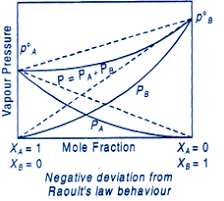# Liquids A and B form an ideal solution in the entire composition range . At 350 K , the vapor pressures of pure A and pure B are , respectively . The composition of the vapor in equilibrium with a solution containing 40 mol percent of A at this temperature is :Option 1)Option 2)Option 3)Option 4)

Mole Fraction -

-

Vapour Pressure -

It is defined as pressure exerted by vapours on liquid surface at equilibrium and condensation.

-

Negative deviation from Raoult's Law behaviour -

When the pressure exerted by vapours of mixture is less than that in case of ideal behaviour.- wherein

Factors affecting vapour pressure -

- wherein

It depends only on temperature and nature of liquid.

as we have learned in partial pressure

we know that

Option 1)

Option 2)

Option 3)

Option 4)

Exams
Articles
Questions